# High School Math : How to find an angle in a parallelogram

## Example Questions

### Example Question #6 : Parallelograms

In the parallellogram, what is the value of?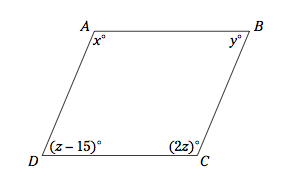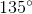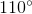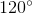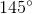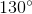Explanation:

Opposite angles are equal, and adjacent angles must sum to 180.

Therefore, we can set up an equation to solve for z:

(z – 15) + 2z = 180

3z - 15 = 180

3z = 195

z = 65

Now solve for x:

2= x = 130°